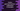# Python gamma() function explanation with example## What is the gamma function in python :

gamma() _function is defined in Python _Math module. It takes one argument and calculates the gamma value for that argument. The gamma function is defined as below :

``gamma(x) = factorial(x - 1)``

That means the gamma of a number is equal to the factorial of number - 1.

## Syntax of gamma function :

The syntax of the gamma function is as below :

``Math.gamma(x)``

Where, x _is the number we are calculating the gamma value. This number should be always _positive. If it is negative, it will throw one ValueError. If the argument is not a number, it will throw one TypeError. Note that gamma() will return one output if the argument is positive or even negative decimal.

### Example of gamma :

Let’s try to check this function with different examples :

``````import math

print("Gamma for 5 is : {}".format(math.gamma(5)))
print("Gamma for 2.5 is : {}".format(math.gamma(2.5)))
print("Gamma for -8.3 is : {}".format(math.gamma(-8.3)))``````

It will print the below output :

``````Gamma for 5 is : 24.0
Gamma for 2.5 is : 1.3293403881791372
Gamma for -8.3 is : -5.040817747151161e-05``````

Now let’s try to compare gamma with its equivalent factorial for different numbers :

``````import math

print("Gamma for 5 is : {}".format(math.gamma(5)))
print("Factorial for 4 is : {}".format(math.factorial(4)))
print("----------------")
print("Gamma for 18 is : {}".format(math.gamma(18)))
print("Factorial for 17 is : {}".format(math.factorial(17)))
print("----------------")
print("Gamma for 15 is : {}".format(math.gamma(15)))
print("Factorial for 14 is : {}".format(math.factorial(14)))
print("----------------")
print("Gamma for 10 is : {}".format(math.gamma(10)))
print("Factorial for 9 is : {}".format(math.factorial(9)))``````

It will print :

``````Gamma for 5 is : 24.0
Factorial for 4 is : 24
----------------
Gamma for 18 is : 355687428096000.0
Factorial for 17 is : 355687428096000
----------------
Gamma for 15 is : 87178291200.0
Factorial for 14 is : 87178291200
----------------
Gamma for 10 is : 362880.0
Factorial for 9 is : 362880``````

As you can see that the gamma of a number is equal to the factorial(number - 1).